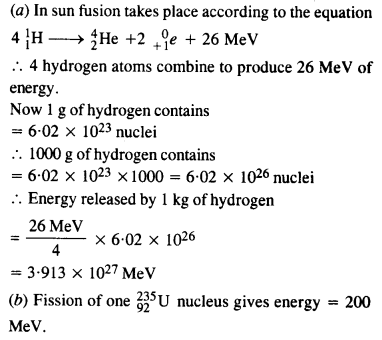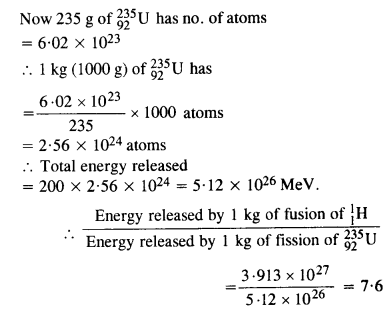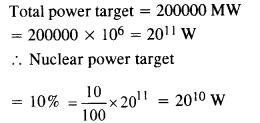## NCERT Solutions for Class 12 Physics Chapter 13 Nuclei

NCERT Solutions for Class 12 Physics Chapter 13 Nuclei are part of NCERT Solutions for Class 12 Physics. Here we have given. NCERT Solutions for Class 12 Physics Chapter 13 Nuclei

 Board CBSE Textbook NCERT Class Class 12 Subject Physics Chapter Chapter 13 Chapter Name Nuclei Number of Questions Solved 31 Category NCERT Solutions

Question 1.
(a) Two stable isotopes of lithium $$_{ 3 }^{ 6 }{ Li }$$ and $$_{ 3 }^{ 7 }{ Li }$$ have respective abundance of 7.5% and 92.5%. These isotopes have masses 6.01512 u and 7.01600 u respectively. Find the atomic weight of lithium.
(b) Boron has two stable isotopes $$_{ 5}^{ 10 }{ Li }$$ and $$_{5 }^{ 11 }{ Li }$$ .Their respective masses are 10.01294 u and 11.00931 u and the atomic weight of boron is 10.811 u. Find the abundances of $$_{ 5 }^{ 10 }{ Li }$$ and
$$_{5 }^{ 11 }{ Li }$$
(a) Atomic weight of lithium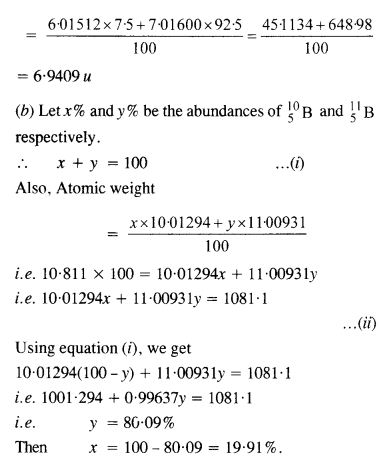Question 2.

The three stable isotopes of neon :$$_{ 20}^{ 10 }{ Ne }$$ and $$_{ 22}^{ 10 }{ Ne }$$  have respective abundance of 90.51%, 0.27% and 9.22%. The atomic masses of three isotopes are 19.99 u, 20.99 u and 21.99 u, respectively. Obtain the average atomic mass of neon.Question 3.
Obtain the binding energy of a nitrogen nucleus ($$_{ 7}^{ 14 }{ N }$$) from the following data :
mH = 1.00783 u
mn = 1.00867 u
mn = 14.00307 uQuestion 4.
Obtain the binding energy of the nuclei $$_{ 26 }^{ 56 }{ Fe }$$ and
in units of $$_{ 83 }^{ 209 }{ Bi}$$ from the following data:
mH =1007825u
mn =1008665u
m ($$_{ 26 }^{ 56 }{ Fe }$$)= 55.934939 u
m ($$_{83}^{209 }{ Bi}$$)
Which nucleus has greater binding energy per nucleon?Question 5.
A given coin has a mass of 3.0 g. Calculate the nuclear energy that would be required to separate all the neutrons and protons from each other. For simplicity assume that the coin is entirely made of $$_{ 29 }^{ 63 }{ Cu }$$ atoms (of mass 62.92960 u). The masses of proton and neutron are 1.00783 u and 1.00867 u, respectively.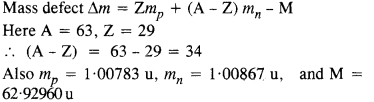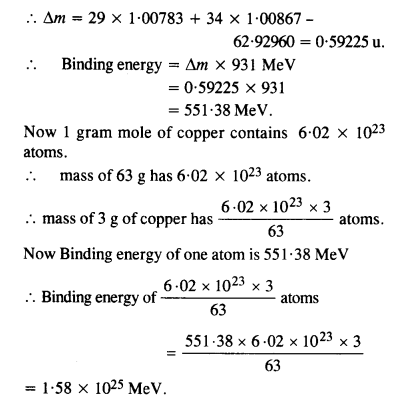Question 6.
Write nuclear equations for :
(a) the α-decay of $$_{ 86 }^{226 }{ Ra}$$
(b) the β-decay of $$_{ 15 }^{ 32 }{ p }$$
(c) the β+-decay of $$_{ 6 }^{ 11 }{ p }$$Question 7.
A radioactive isotope has a half-life of T years. After how much time is its activity reduced to 3.125% of its original activity (b) 1% of original value ?Question 8.
The normal activity of living carbon-containing matter is found to be about 15 decays per minute for every gram of carbon. This activity arises from the small proportion of radioactive $$_{ 6 }^{ 14 }{ C }$$ present with the stable carbon isotope
$$_{ 12 }^{ 6 }{ C }$$ When the organism is dead, its interaction with the atmosphere (which maintains the above equilibrium activity) ceases and its activity begins to drop. From the known half-life (5730 years) of $$_{ 6 }^{ 14 }{ C }$$ , and the measured activity, the age of the specimen can be approximately estimated. This is the principle of $$_{ 6 }^{ 14 }{ C }$$ dating used in archaeology. Suppose a specimen from Mohenjodaro gives an activity of 9 decays per minute per gram of carbon. Estimate the approximate age of the Indus-Valley civilisation.Question 9.
Obtain the amount of $$_{ 27 }^{ 60 }{ Co }$$ necessary to provide a radioactive source of 8.0 mCi strength. The half­ life of $$_{ 27 }^{ 60 }{ Co }$$ is 5.3 years.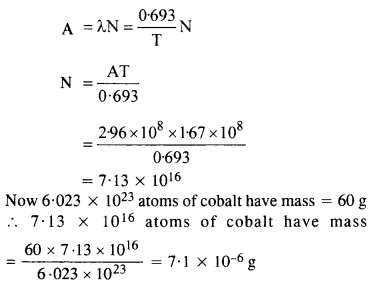Question 10.
The half-life of $$_{ 38 }^{ 90 }{ Sr }$$ is 28 years. What is the disintegration rate of 15 mg of this isotope?Question 11.
Obtain approximately the ratio of the nuclear radii of the gold isotope $$_{ 79 }^{ 197}{ Au }$$ and silver isotope $$_{47}^{107 }{ Au }$$.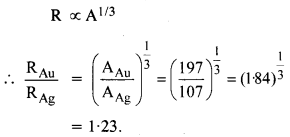Question 12.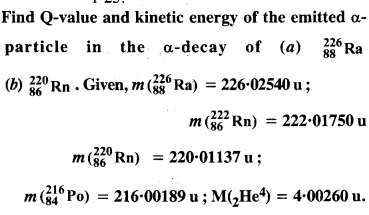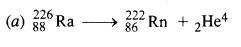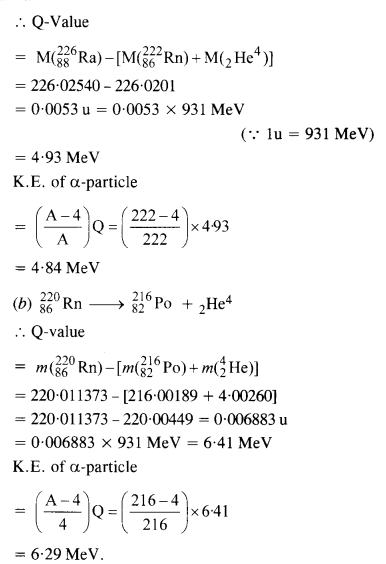Question 13.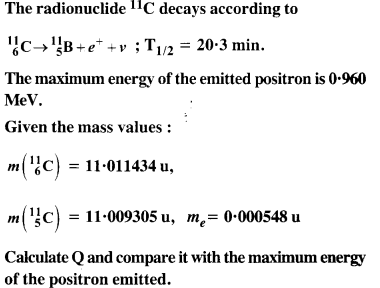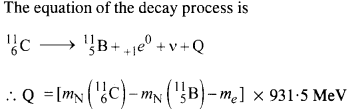Here mN stands for the nuclear mass of the element or particle. In order to express the Q value in terms of the atomic masses, 6 me mass has to be subtracted from the atomic mass of $$_{6}^{11 }{ Au }$$ and 5 me mass has to be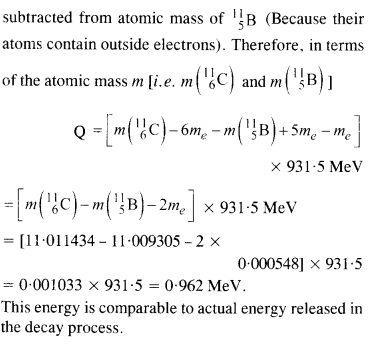Question 14.
The nucleus $$_{ 10 }^{ 23 }{ Ne}$$ decays by β~ emission. Write down the p-decay equation and determine the maximum kinetic energy of the electrons emitted. Given that:
m($$_{ 10 }^{ 23 }{ Sr }$$) = 22.994466 u
m($$_{ 11 }^{ 23 }{ Sr }$$) = 22.989770 u.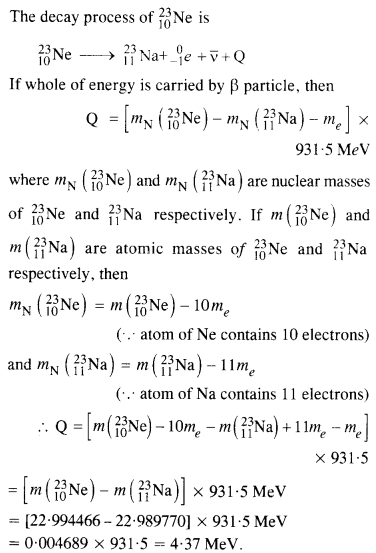Question 15.
The Q value of a nuclear reaction A + b ⇒ C + d is defined by [Q = mA + mb-mc– md] c2 where the masses refer to nuclear rest masses. Determine from the given data whether the following reactions are exothermic or endothermic.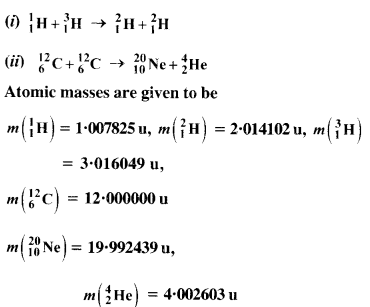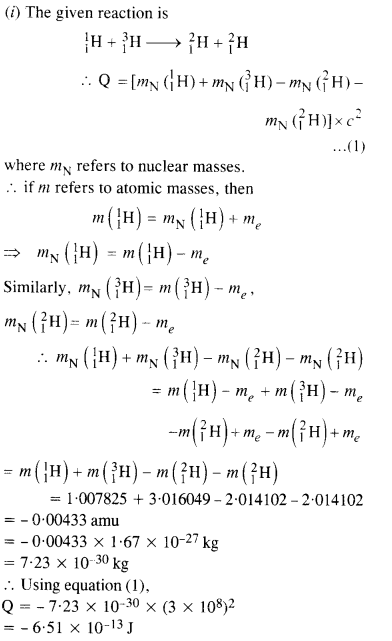Question 16.
Suppose, we think of fission of a $$_{ 26}^{ 56 }{ Fe}$$ nucleus into two equal fragments, if $$_{ 13}^{ 28 }{ Al }$$. Is the fission energetically possible ? Argue by working out Q of the process. Given, m ($$_{ 26}^{ 56 }{ Fe}$$) = 55.93494 u and m ($$_{ 13}^{ 28 }{ Al }$$)= 27.98191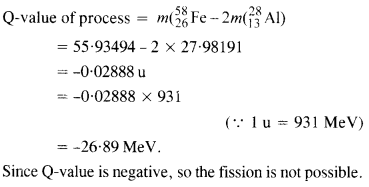Question 17.
The fission properties of $$_{ 94}^{ 239 }{ Pu}$$ are very similar to those of $$_{ 92}^{ 235 }{ u}$$u. The average energy released per fission is 180 MeV. How much energy, in MeV, is released if all the atoms in 1 kg of pure
$$_{94}^{ 239 }{ Pu}$$ undergo fission ?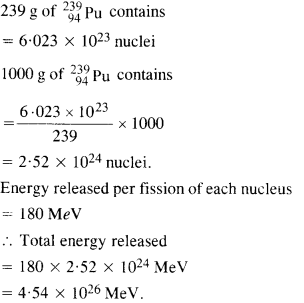Question 18.
A 1000 MW fission reactor consumes half of its fuel in 5.00 y. How much
$$_{ 92}^{ 235 }{ u}$$ did it contain initially ? Assume that all the energy generated arises from the fission of $$_{92}^{ 235 }{ u}$$ and that this nuclide is consumed by the fission process.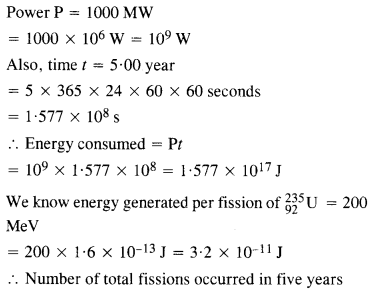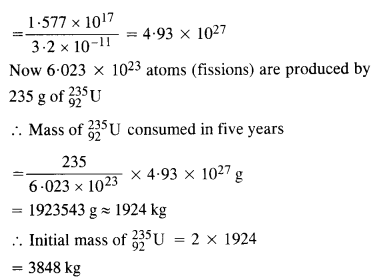Question 19.
How long an electric lamp of 100 W can be kept glowing by fusion of 2.0 kg of deuterium ? The fusion reaction can be taken as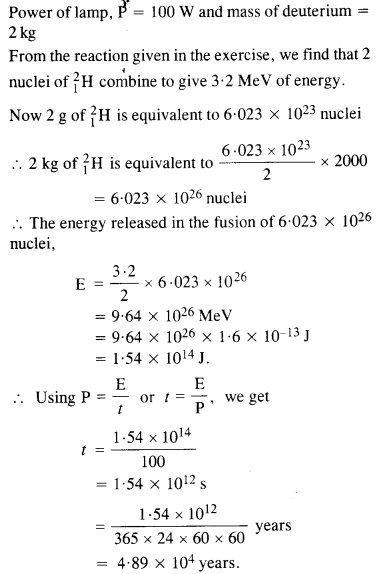Question 20.
Calculate the height of Coulomb barrier for the head on collision of two deuterons. The effective radius of deuteron can be taken to be 2.0 fm.
The initial mechanical energy E of the two deutrons before collision is given by
E = 2 K.E.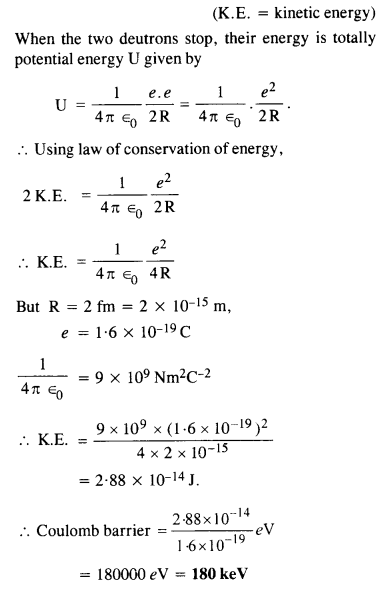Question 21.
From the relation R = RA1/3, where R is a constant and A is the mass number of a nucleus, show that nuclear matter density is nearly constant (i.e. independent of A)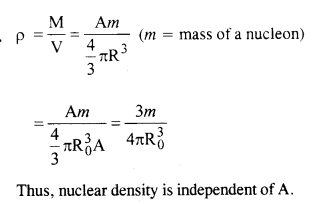Question 22.
For the β+ (positron) emission from a nucleus, there is another competing process known as electron capture (electron from an inner orbit, say, the K- shell, is captured by the nucleus and a neutrino is emitted).Show that if β+ emission is energetically allowed, electron capture is necessarily allowed but not vice-versa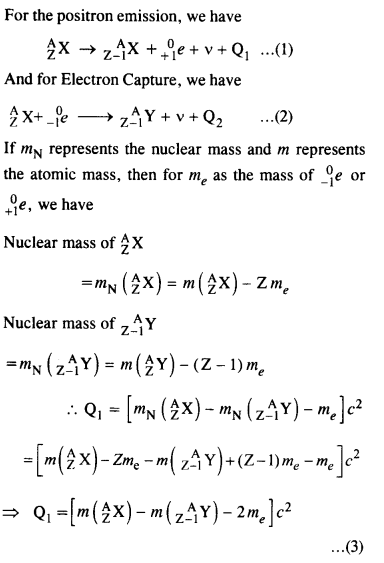Question 23.
In a Periodic Table the average atomic mass of magnesium is given as 24.312 u. The average value is based on their relative natural abundance on Earth. The three isotopes and their masses are $$_{ 12}^{ 24 }{ Mg}$$ (23.98504u), ? $$_{12}^{ 25 }{ Mg}$$ (24.98584) and $$_{ 12}^{ 26 }{ Mg}$$ (25.98259u). The natural abundance of $$_{ 12}^{ 24 }{ Mg}$$ is 78.99% by mass. Calculate the abundances of the other two isotopes.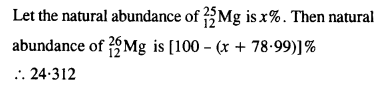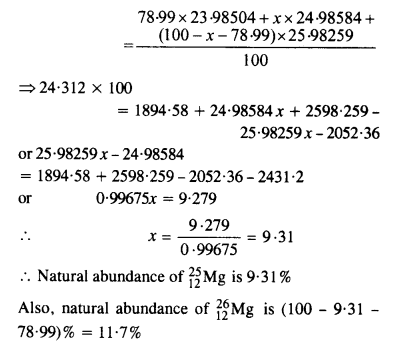Question 24.
The neutron separation energy is defined as the energy required to remove a neutron from the nucleus. Obtain the neutron separation energies of the nuclei $$_{ 12}^{ 24 }{ Ca}$$ and $$_{ 13}^{ 27 }{ Al}$$ from the following data :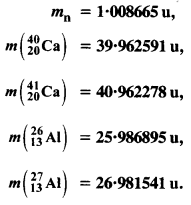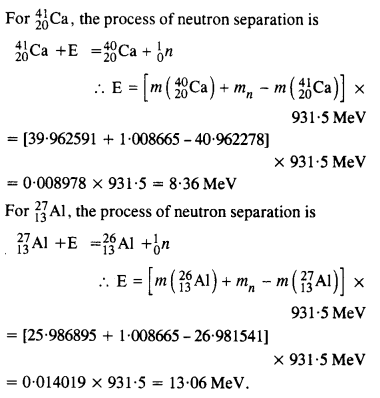Question 25.Question 26.
Under certain circumstances, a nucleus can decay by emitting a particle more massive than an α-particle. Consider the following decay processes :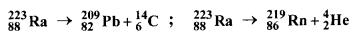(a) Calculate the Q values for these decays and determine that both are energetically possible.
(b) The Coulomb barrier height for α-particle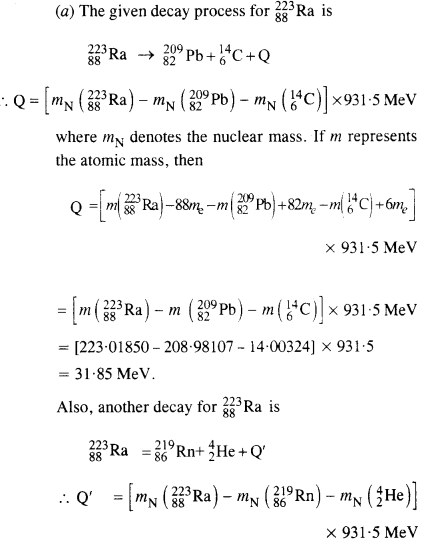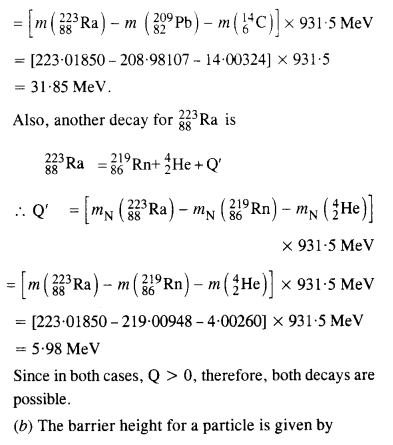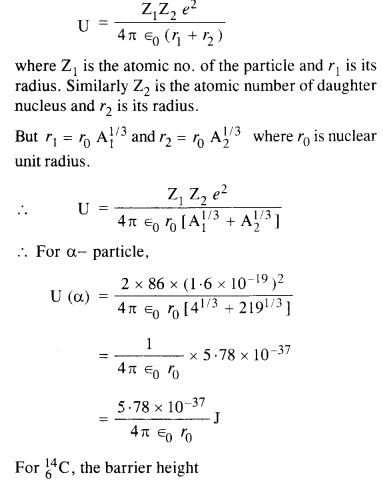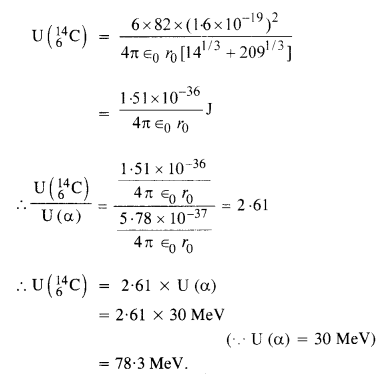Question 27.
Consider the fission of $$_{ 92}^{ 239}{u}$$ by fast neutrons. In one fission event, no neutrons are emitted and the final stable end products, after the beta-decay of the primary fragments, are $$_{58}^{ 140}{Ce}$$ and $$_{44}^{ 99}{Ru}$$. Calculate Q for this fission process. The relevant atomic and particle masses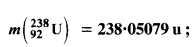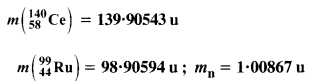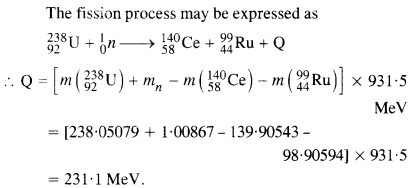Question 28.
Consider the D-T reaction (deuterium-tritium-fusion) given in eqn. :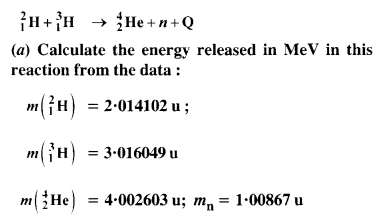(b) Consider the radius of both deuterium and tritium to be approximately 1.5 fm. What is the kinetic energy needed to overcome the Coulomb repulsion? To what temperature must the gases be heated to initiate the reaction?
From the equation given in the question,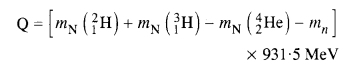mN refers to the nuclear mass of the element given in the brackets and mn = mass of the neutron. If in represents the atomic mass, then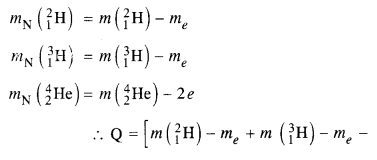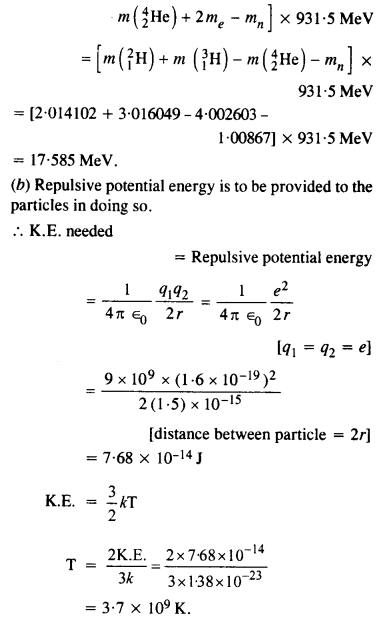Question 29.
Obtain the maximum kinetic energy of p-particles and the radiation frequencies to y decay in the following decay scheme. You are given that
m (198Au) = 197.968233 u
m (198Hg) = 197.966760 u
The total energy released for the transformation of $$_{79}^{ 198}{Au}$$ to $$_{80}^{ 198}{u}$$ can be found by considering the energies of ϒ-rays. We first find the frequencies of the ϒ-rays emitted.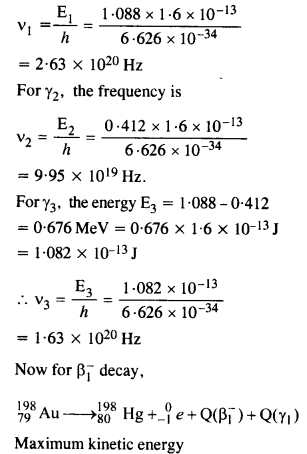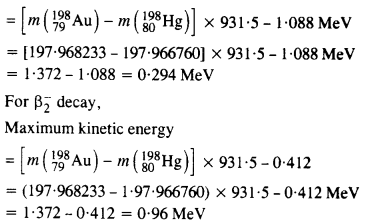Question 30.

Calculate and compare the energy released by (a) fusion of 1.0 kg of hydrogen deep within the sun and (b) the fission of 1.0 kg of 235U in a fission reactor.